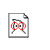# Automatic Synthesis of Electrical Circuits Containing a Free Variable using Genetic Programming

Created by W.Langdon from gp-bibliography.bib Revision:1.5776

@InProceedings{Koza:2000:GECCO,
• author = "John R. Koza and Martin A. Keane and Jessen Yu and William Mydlowec",
• title = "Automatic Synthesis of Electrical Circuits Containing a Free Variable using Genetic Programming",
• pages = "477--484",
• year = "2000",
• publisher = "Morgan Kaufmann",
• booktitle = "Proceedings of the Genetic and Evolutionary Computation Conference (GECCO-2000)",
• editor = "Darrell Whitley and David Goldberg and Erick Cantu-Paz and Lee Spector and Ian Parmee and Hans-Georg Beyer",
• address = "Las Vegas, Nevada, USA",
• publisher_address = "San Francisco, CA 94104, USA",
• month = "10-12 " # jul,
• keywords = "genetic algorithms, genetic programming",
• ISBN = "1-55860-708-0",
• URL = "http://gpbib.cs.ucl.ac.uk/gecco2000/GP147.pdf",
• URL = "http://gpbib.cs.ucl.ac.uk/gecco2000/GP147.ps",
• URL = "http://www.genetic-programming.com/jkpdf/gecco2000parameterizedfilter.pdf",
• URL = "http://citeseer.ist.psu.edu/495857.html",
• abstract = "A mathematical formula containing one or more free variables is {"}general{"} in the sense that it represents the solution to all instances of a problem (instead of just the solution of a single instance of the problem). For example, the familiar formula for solving a quadratic equation contains free variables representing the coefficients of the to-be-solved equation. This paper demonstrates, using an illustrative problem, that genetic programming can automatically create the design for both the topology and component values for an analog electrical circuit in which the value of each component in the evolved circuit is specified by a mathematical expression containing a free variable. That is, genetic programming is used to evolve a general parameterized circuit that satisfies the problem's high-level requirements. The evolved circuit has been cross-validated on unseen values of the free variable.",
• notes = "A joint meeting of the ninth International Conference on Genetic Algorithms (ICGA-2000) and the fifth Annual Genetic Programming Conference (GP-2000) Part of \cite{whitley:2000:GECCO}",
}

Genetic Programming entries for John Koza Martin A Keane Jessen Yu William J Mydlowec